### Home > CALC > Chapter 9 > Lesson 9.1.3 > Problem9-45

9-45.
1. Determine whether each of the infinite series converges or diverges. Explain each answer briefly. If the series converges, find its exact sum. Homework Help ✎

1.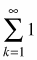2.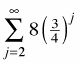3.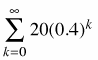4.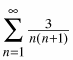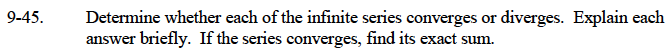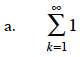What is 1 + 1 + 1 + 1 + ... ?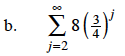This is a geometric sequence with a ratio of 3/4.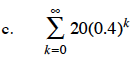This is also a geometric sequence. What is the ratio? (See part (b).)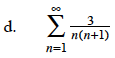This can be written as a telescoping sum:

$S=3\bigg(\frac{1}{2}+\frac{1}{6}+\frac{1}{12}+...\bigg)$

$\text{ }=3\bigg(\Big(1-\frac{1}{2}+\Big(\frac{1}{2}-\frac{1}{3}\Big)+\Big(\frac{1}{3}-\frac{1}{4}\Big)+...\bigg)$